• 定义复杂结构体—包括嵌套结构体数组，并显示结构体所占字节数。
• 讲解区分结构体与结构体数组：结构体的引出、结构体变量的定义和引用、结构体数组
• 结构体数组 结构体数组格式：声明变量类型 数组名称[数组长度] //定义一个结构体数组名为a,它里面包含有5个元素，每一个元素都是一个结构体变量 Wor a={}; ...
结构体数组
结构体数组的定义：
结构体数组格式：声明变量类型 数组名称[数组长度]
//定义一个结构体数组名为a,它里面包含有5个元素，每一个元素都是一个结构体变量
Wor a={};

#include<stdio.h>
typedef struct worker{
char id;
char name;
char sex;
int pay;
}WOR;
void main(){
WOR a=
{
{"0001","陈文杰","男",1200},
{"0001","张三丰","女",500},
{"0001","张四丰","女",1200}
}
}

对比记忆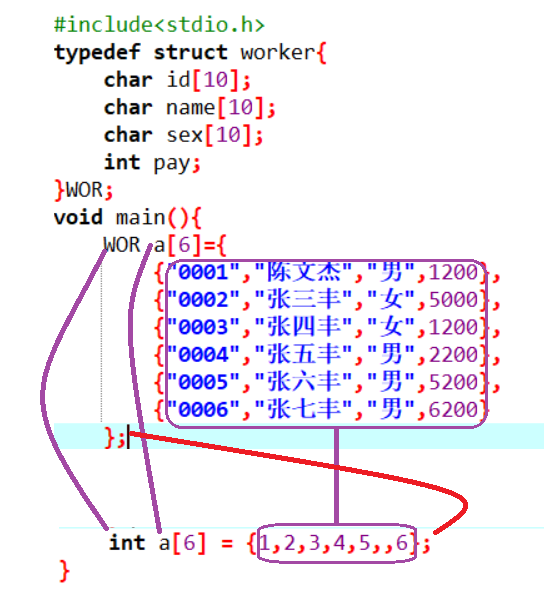结构体数组的输出
#include<stdio.h>
typedef struct worker{
char id;
char name;
char sex;
int pay;
}WOR;
void main(){
WOR a={
{"0001","陈文杰","男",1200},
{"0002","张三丰","女",5000},
{"0003","张四丰","女",1200},
{"0004","张五丰","男",2200},
{"0005","张六丰","男",5200},
{"0006","张七丰","男",6200}
};
int i;
for(i=0;i<6;i++){
printf("%s,%s,%s,%d\n",a[i].id,a[i].name,a[i].sex,a[i].pay);
}
}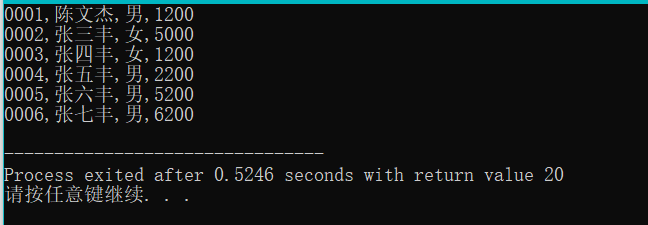结构体对象中的a[i]就代表一个对象，它已经不再是一个值了 所以我们应该用a[ii].成员名
改进以上程序
#include<stdio.h>
typedef struct worker{
char id;
char name;
char sex;
int pay;
}WOR;
void output(WOR a[],int n){//次函数是用来输出长度为n的工人数组的信息
int i;
printf("工号\t姓名\t性别\t年龄\n");
for(i=0;i<6;i++){
printf("%s\t%s\t%s\t%d\n",a[i].id,a[i].name,a[i].sex,a[i].pay);
}
}
void main(){
WOR a={
{"0001","陈文杰","男",1200},
{"0002","张三丰","女",5000},
{"0003","张四丰","女",1200},
{"0004","张五丰","男",2200},
{"0005","张六丰","男",5200},
{"0006","张七丰","男",6200}
};
output(a,6);
}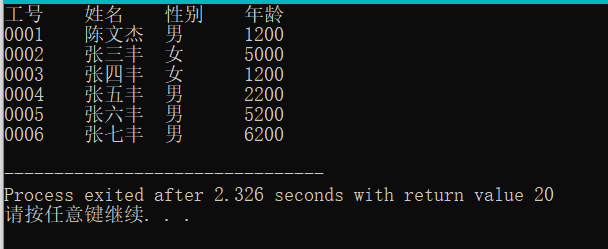结构体的应用
案例一、
1、编写一个函数，它的目的是为了输出所有性别为男的的职工信息 算法分析： 1)这个函数它不用返回值因为该函数是为了完成输出 2）该函数要处理很多职工，就需要一个数组，数组的类型为职工类型 注意：在C语言当中字符串不能直接比较是否相同，要通过strcmp这个函数来比较其两个字符串是否相同
#include<stdio.h>
#include<string.h>
typedef struct worker{
char id;
char name;
char sex;
int pay;
}WOR;
void QueryWorker(WOR a[],int n){//次函数是用来输出长度为n的工人数组的信息
int i;
printf("全部员工：\n");
printf("工号\t姓名\t性别\t年龄\n");
for(i=0;i<6;i++){
printf("%s\t%s\t%s\t%d\n",a[i].id,a[i].name,a[i].sex,a[i].pay);
}
printf("所有男员工：\n");
printf("工号\t姓名\t性别\t年龄\n");
for(i=0;i<6;i++){
if(strcmp(a[i].sex,"男")==0){
printf("%s\t%s\t%s\t%d\n",a[i].id,a[i].name,a[i].sex,a[i].pay);
}
}
printf("所有女员工：\n");
printf("工号\t姓名\t性别\t年龄\n");
for(i=0;i<6;i++){
if(strcmp(a[i].sex,"女")==0){
printf("%s\t%s\t%s\t%d\n",a[i].id,a[i].name,a[i].sex,a[i].pay);
}
}
}
void main(){
WOR a={
{"0001","陈文杰","男",1200},
{"0002","张三丰","女",5000},
{"0003","张四丰","女",1200},
{"0004","张五丰","男",2200},
{"0005","张六丰","男",5200},
{"0006","张七丰","男",6200}
};
QueryWorker(a,6);
}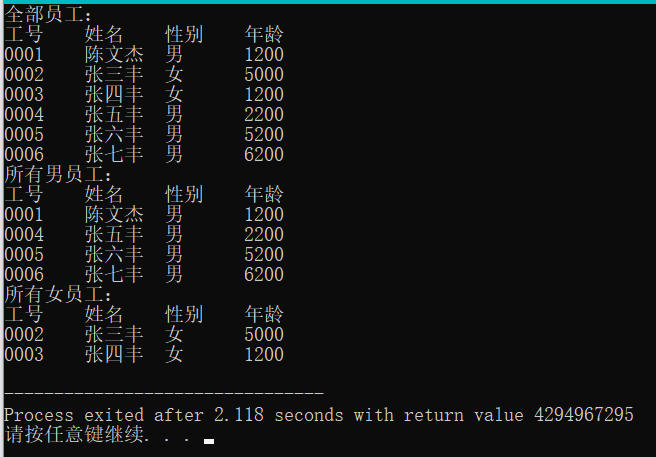2、能够输出指定性别的职工
#include<stdio.h>
typedef struct worker{
char id;
char name;
char sex;
int pay;
}WOR;
void QueryBySex(WOR a[],int n,char b[]){//次函数是用来输出长度为n的工人数组的信息
int i;

printf("工号\t姓名\t性别\t年龄\n");
for(i=0;i<6;i++){
if(strcmp(a[i].sex,b)==0){
printf("%s\t%s\t%s\t%d\n",a[i].id,a[i].name,a[i].sex,a[i].pay);
}
}

}
void main(){
WOR a={
{"0001","陈文杰","男",1200},
{"0002","张三丰","女",5000},
{"0003","张四丰","女",1200},
{"0004","张五丰","男",2200},
{"0005","张六丰","男",5200},
{"0006","张七丰","男",6200}
};
char b;
printf("请输入您要查询的性别 :\n");
scanf("%s",b);
QueryBySex(a,6,b);
}

案例二、
让用户输入一个工号，然后在一个结构体数组当中去查找有没有这个工人，如果有则输出这个工人的信息，如果没有则找不到此人
#include<stdio.h>
typedef struct worker{
char id;
char name;
char sex;
int pay;
}WOR;

void FindById(WOR a[],int n,char id[]){
//我们要访问数组里面每一个工人，如果发现了一个工人，就退出循环。如果找不到就输出找不到此人
int i;
for(i=0;i<n;i++){
if(strcmp(a[i].id,id)==0){//说明第i个工人就是我们要找的职工
break;//强制终止循环
}
}

//在退出循环之后，再来检查i与n的关系
if(i<n){//说明循环在中途就break,发现了职工
printf("%s\t%s\t%s\t%d\n",a[i].id,a[i].name,a[i].sex,a[i].pay);
}
else//找不到职工
{
printf("找不到此人");
}
}

void main(){
WOR a={
{"0001","陈文杰","男",1200},
{"0002","张三丰","女",5000},
{"0003","张四丰","女",1200},
{"0004","张五丰","男",2200},
{"0005","张六丰","男",5200},
{"0006","张七丰","男",6200}
};
char b;
printf("请输入您要查询的id :\n");
gets(b);
FindById(a,6,b);
}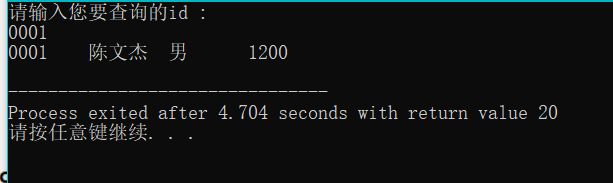案例三、
求出所有职工中最高工资那个职工的信息 注意：这里有一个知识点：成员比较整体赋值
#include<stdio.h>
#include<string.h>
typedef struct worker{
char id;
char name;
char sex;
int pay;
}WOR;
void max(WOR a[],int n){
WOR m=a;
int i;
for(i=0;i<n;i++){
if(m.pay<a[i].pay){//如果m的工资小于a[i]的工资，就把m赋值给a[i]
m=a[i];
}
}
printf("%s\t%s\t%s\t%d\n",m.id,m.name,m.sex,m.pay);
//假设第一个工人是最高工资的那个工人
}
void main(){
WOR a={
{"0001","陈文杰","男",1200},
{"0002","张三丰","女",7000},
{"0003","张四丰","女",1200},
{"0004","张五丰","男",2200},
{"0005","张六丰","男",5200},
{"0006","张七丰","男",6200}
};
max(a,6);
}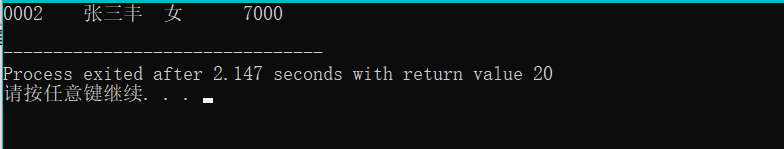上方程序改进 输出所有工资大于平均工资的职工 1、必须要先求出平均 2、在访问每一个职工，如果发现这个职工的工资要小于平均工资的话，就输出这个职工的信息。
#include<stdio.h>
#include<string.h>
typedef struct worker{
char id;
char name;
char sex;
int pay;
}WOR;
void QueryByAver(WOR a[],int n){
int i;
float aver;
int sum = 0;
//求出总工资
for(i=0;i<n;i++){
sum+=a[i].pay;
}
//求出平均工资aver
aver =(float)sum/n;
printf("平均工资为%f\n",aver);
printf("大于平均工资为：\n");
//访问每一个职工
for(i=0;i<n;i++){
if(a[i].pay>=aver){//如果发现职工的工资大于等于平局工资，就输出对应职工的信息
printf("%s\t%s\t%s\t%d\n",a[i].id,a[i].name,a[i].sex,a[i].pay);
}
}
}
void main(){
WOR a={
{"0001","陈文杰","男",3200},
{"0002","张三丰","女",7000},
{"0003","张四丰","女",1200},
{"0004","张五丰","男",2200},
{"0005","张六丰","男",5200},
{"0006","张七丰","男",6200}
};
QueryByAver(a,6);
}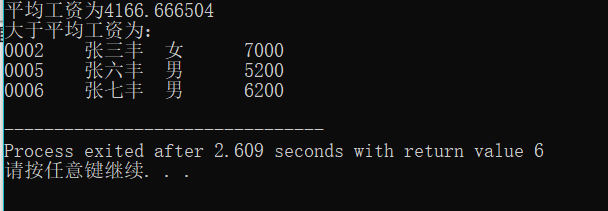算法变换
#include<stdio.h>
#include<string.h>
typedef struct stud{//定义了一个结构体
char Id;//学号
char Name;//姓名
int China;//语文成绩
int Math;//数学成绩
int English;//英语成绩
}STU;
//编写一个函数，它的目的是为了输出所有学生的总分与平均分
void fun(STU a[],int n){
int sum=0,i;
printf("学号\t姓名\t语文\t数学\t英语\t总分\t平局分\n");
//要访问里面的每一个学生
for(i=0;i<n;i++){
//对于一个学生a[i]而言我们要来输出它的信息
printf("%s\t%s\t%d\t%d\t%d\t%d\t%.1f\n",
a[i].Id,a[i].Name,a[i].China,a[i].Math,a[i].English,
a[i].China+a[i].Math+a[i].English,
(a[i].China+a[i].Math+a[i].English)/3.0);
sum = a[i].China+a[i].Math+a[i].English;
}
}
void main(){
STU a={
{"S1","张一军",127,150,100},
{"S2","张二军",75,85,89},
{"S3","张三军",67,58,86},
{"S4","张四军",120,101,87},
{"S5","张五军",136,100,82},
{"S6","张六军",141,52,83},
{"S7","张七军",125,85,81},
{"S8","张八军",136,65,79}
};
fun(a,8);
}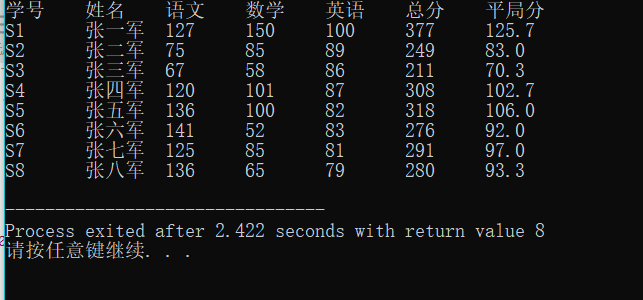案例变换： 注意字符串也不能够直接赋值，要通过一个函数来对字符串进行赋值 strcpy:通过这个函数才可以给字符串进行赋值
#include<stdio.h>
#include<string.h>
typedef struct stud{//定义了一个结构体
char Id;//学号
char Name;//姓名
int China;//语文成绩
int Math;//数学成绩
int English;//英语成绩
}STU;
//编写一个函数，求出每一个学生的优秀科目，目的不是输出分数，而是要输出对应科目的名称
void Perferct(STU a[],int n){
int sum=0,i;
char per;
int maxscore;//用来确保最优科目的分数
//要输出标题
printf("学号\t姓名\t语文\t数学\t英语\t最优秀科目\n");
//要访问里面的每一个学生，对于第i学生要确认它的最优科目名称（语文，数学，英语）
//科目名称是字符串，所以要先定义一个字符串来确保保存最优科目名称
for(i=0;i<n;i++){
maxscore=a[i].China;//认为第i个学生的语文是最优秀的
strcpy(per,"语文");
if(maxscore<a[i].Math){
maxscore = a[i].Math;
strcpy(per,"数学");
}
if(maxscore<a[i].English){
maxscore = a[i].Math;
strcpy(per,"英语");
}
printf("%s\t%s\t%d\t%d\t%d\t%s\n",
a[i].Id,a[i].Name,a[i].China,a[i].Math,a[i].English,per);
}
}

void main(){
STU a={
{"S1","张一军",127,150,100},
{"S2","张二军",75,85,89},
{"S3","张三军",67,58,86},
{"S4","张四军",120,101,87},
{"S5","张五军",136,100,82},
{"S6","张六军",141,52,83},
{"S7","张七军",125,85,81},
{"S8","张八军",136,65,79}
};
Perferct(a,8);
}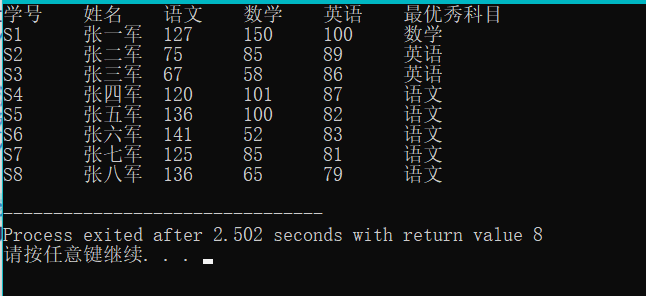展开全文c语言 程序设计 指针 编程语言
• 1.结构体 结构体是用户自定义的可用的数据类型，它允许您存储不同类型的数据项。 2.结构体定义 以学生的基本信息为例，包括四个变量...（2）定义了一个结构体STU的同时定义了两个结构体STU的变量stu2，stu3。 struct
1.结构体 结构体是用户自定义的可用的数据类型，它允许您存储不同类型的数据项。 2.结构体的定义 以学生的基本信息为例，包括四个变量：姓名、年龄、性别、学号。 （1）定义了一个结构体STU
struct STU
{
char name;
int age;
char sex;
char num;
};

定义结构体变量:
struct STU stu1,stu2;

（2）定义了一个结构体STU的同时定义了两个结构体STU的变量stu2，stu3。
struct STU
{
char name;
int age;
char sex;
char num;
}stu1,stu2;

此时还可以继续定义STU结构体变量如：
struct STU stu3;

(3)定义没有给出该结构体的名称,直接定义结构体变量stu1,stu2后就不能再继续定义该类型的变量。
struct
{
char name;
int age;
char sex;
char num;
}stu1,stu2;

(4)在第一种结构定义的基础上加了关键字typedef,但是因为没有给出别名,直接用STU定义变量是不行的。如STU stu1,但struct STU stu1可行。
typedef struct STU
{
char name;
int age;
char sex;
char num;
};

定义结构体变量：
struct STU stu1,stu2;

(5)第五种结构体定义在第四种结构体定义的基础上加上了别名stu1，可以用stu1定义STU的结构体变量,如stu1 stu2。用STU不能直接定义，需要在前面加struct，如struct STU stu3。
typedef struct STU
{
char name;
int age;
char sex;
char num;
}stu1;

定义结构体变量：
stu1 stu2;
struct STU stu3;

(6)第六种结构体定义在第五种的基础上减去了结构体名，但是若是直接使用stu1来定义该结构体类型的变量也是可以的。如stu1 stu2。
typedef struct
{
char name;
int age;
char sex;
char num;
}stu1;

定义结构体变量：
stu1 stu2;

3.结构体数组 （1）
struct STU
{
char name;
int age;
char sex;
char num;
};

定义： struct STU stu; (2)使用typedef关键字1
struct STU
{
char name;
int age;
char sex;
char num;
};
typedef struct STU stu;

定义： stu a; (3)使用typedef关键字2
typedef struct STU
{
char name;
int age;
char sex;
char num;
}stu;

定义： stu a (4)使用typedef关键字3 省去结构体名
typedef struct
{
char name;
int age;
char sex;
char num;
}stu;

定义： stu a
展开全文结构体 struct typedef
• 主要为大家详细介绍了C语言利用结构体数组实现学生成绩管理系统，具有一定的参考价值，感兴趣的小伙伴们可以参考一下
• C++结构体数组定义 C++结构体数组的定义和定义结构体变量的方法相仿，只需声明其为数组即可 struct Student{ //自定义结构体变量 int num;//学号 char sex;//性别 int age;//年龄 }; Student stu;//定义...
C++结构体数组
C++结构体数组与以前介绍过的数值型数组的不同之处在于：每个数组元素都是一个结构体类 型的数据，它们都分别包括各个成员项。
C++结构体数组定义
C++结构体数组的定义和定义结构体变量的方法相仿，只需声明其为数组即可
struct Student{ //自定义结构体变量
int num;//学号
char sex;//性别
int age;//年龄
};
Student stu;//定义Student类型的结构体数组

struct Student{ //自定义结构体变量
int num;//学号
char sex;//性别
int age;//年龄
}stu;//定义Student类型的结构体数组

struct { //自定义结构体变量
int num;//学号
char sex;//性别
int age;//年龄
}stu;//定义Student类型的结构体数组

C++结构体数组初始化
struct Student{ //自定义结构体变量
int num;//学号
char sex;//性别
int age;//年龄
}stu={{1001,'M',21},{1002,'F',18},{1003,'M',19}};

定义结构体数组stu时，也可以不指定元素个数，即写成以下形式：
stu={{1001,'M',21},{1002,'F',18},{1003,'M',19}};

编译时，系统会根据给出初值的结构体常量的个数来确定数组元素的个数。一个结构体常量应包括结 构体中全部成员的值。
经典案例：C++结构体数组使用。
#include<iostream>//预处理
using namespace std;//命名空间
int main()//主函数
{
struct Student{ //自定义结构体变量
int num;//学号
char sex;//性别
int age;//年龄
}stu={{1001,'M',21},{1002,'F',18},{1003,'M',19}};
for(int i=0;i<3;i++)//循环输出结构体数组信息
{
cout<<stu[i].num<<endl;//输出学号
cout<<stu[i].sex<<endl;//输出性别
cout<<stu[i].age<<endl;//输出年龄
cout<<"---------"<<endl;//隔开
}
return 0; //函数返回值为0；
}

执行本程序之后，会输出：
1001
M
21
---------
1002
F
18
---------
1003
M
19
---------

--------------------------------
Process exited after 0.08727 seconds with return value 0
请按任意键继续. . .

C++结构体数组的使用 更多案例可以go公众号：C语言入门到精通
展开全文c++ 编程语言
• 定义结构体数组定义结构体变量的方式类似，请看下面的例子： struct stu{ char *name; //姓名 int num; //学号 int age; //年龄 char group; //所在小组 float score; //成绩 }class; 表示一个班级有5个...
• C语言结构体数组+结构体类型指针+指向...结构体数组定义的方法和结构体变量定义的方法一样，只不过加了一个数组的长度而已 第一种：和结构体类型同时定义，例如： struct student { int sno; char sname; int
上一篇文章：C语言结构体类型定义+结构体变量的定义与使用及其初始化+结构体变量作为函数参数

C语言结构体数组+结构体类型指针+指向结构体数组的指针+typedef类型
结构体数组两种结构体数组的定义形式用sizeof运算符求结构体数组所占字节数结构体数组初始化结构体数组作为函数的参数查找符合条件的人数(一)

结构体类型指针 和 指向结构体数组的指针指向结构体变量的指针的定义利用结构体指针引用结构体变量成员指向结构体数组的指针查找符合条件的人数(二)
typedef类型学生成绩排序修改学生成绩下一篇文章

结构体数组
如果要处理多个类型相同的结构体数据，就需要定义结构体数组
两种结构体数组的定义形式
结构体数组定义的方法和结构体变量定义的方法一样，只不过加了一个数组的长度而已 第一种：和结构体类型同时定义，例如：
struct student
{
int sno;
char sname;
int sage;
char depmt;
float cscore;
}s1;//定义了一个结构体数组，数组名为s1，数组的长度为10

第二种：和结构体类型分开定义，如：
struct student
{
int sno;
char sname;
int sage;
char depmt;
float cscore;
};//不要忘记最后的分号
main()
{
struct student s1;//在主函数中定义结构体数组，数组名为s1，数组长度为10
...
}

用sizeof运算符求结构体数组所占字节数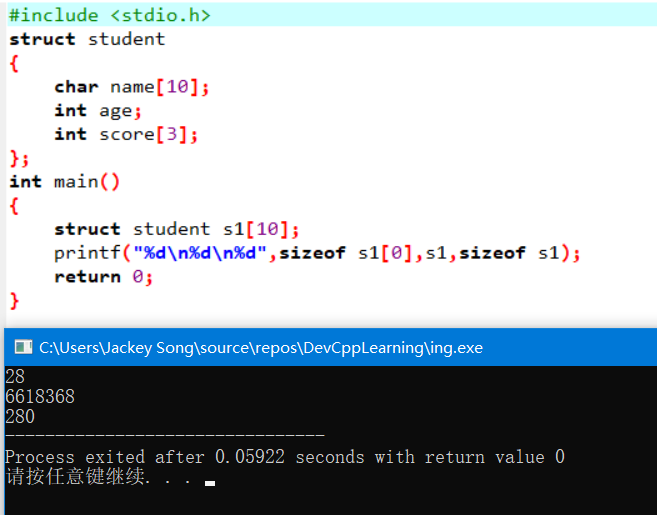char name 占用10个字节，int age 占用4个字节，int score占用3*4=12个字节，加起来总共是26个字节，可是上面输出的是28个字节，多出两个字节。这是因为在编译系统中，结构体类型存在字节边界的要求。而不同编译器字节边界的要求又是不一样的，上面这个案例我使用的是DevC++编译器，然而试验了一番之后，我并没能发现DevC++编译器字节边界要求的规律，反而把我给整懵圈了，如下：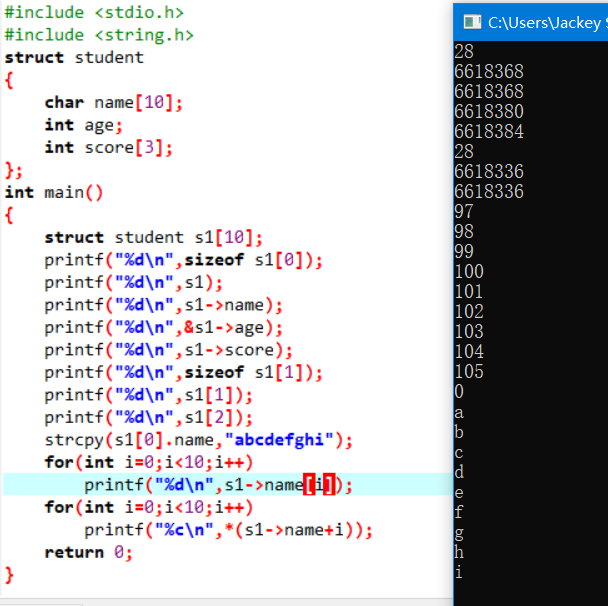虽然它的内存地址分配规律我没能搞懂，但是在使用的时候，依然可以正常用指针来操纵内存地址，进而操纵对应内存地址中的数据。 如下：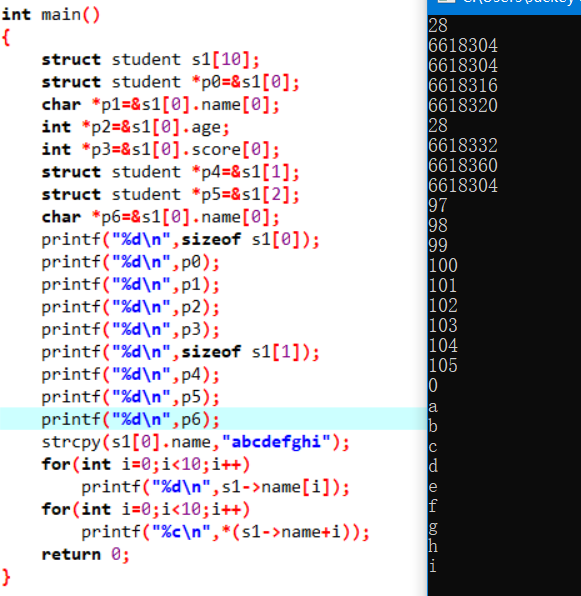结构体中的内存地址分配规律，相对于普通的数据类型的内存地址分配规律，要更加复杂一些，而且不同的编译器也不一样。 在VC编译器中，各成员变量在内存中的起始地址相对于结构体变量(或数组)的起始地址的偏移量，必须为该变量的类型所占字节数的倍数。如果某成员的偏移量不是该类型的整数倍，则VC编译系统会自动填充一定的字节数，以保证字节边界的要求。同时VC为了确保结构体的大小为结构体中所占内存空间最大的成员类型的字节数的倍数，在为最后一个成员变量申请空间后，还会根据需要自动填充空缺的字节。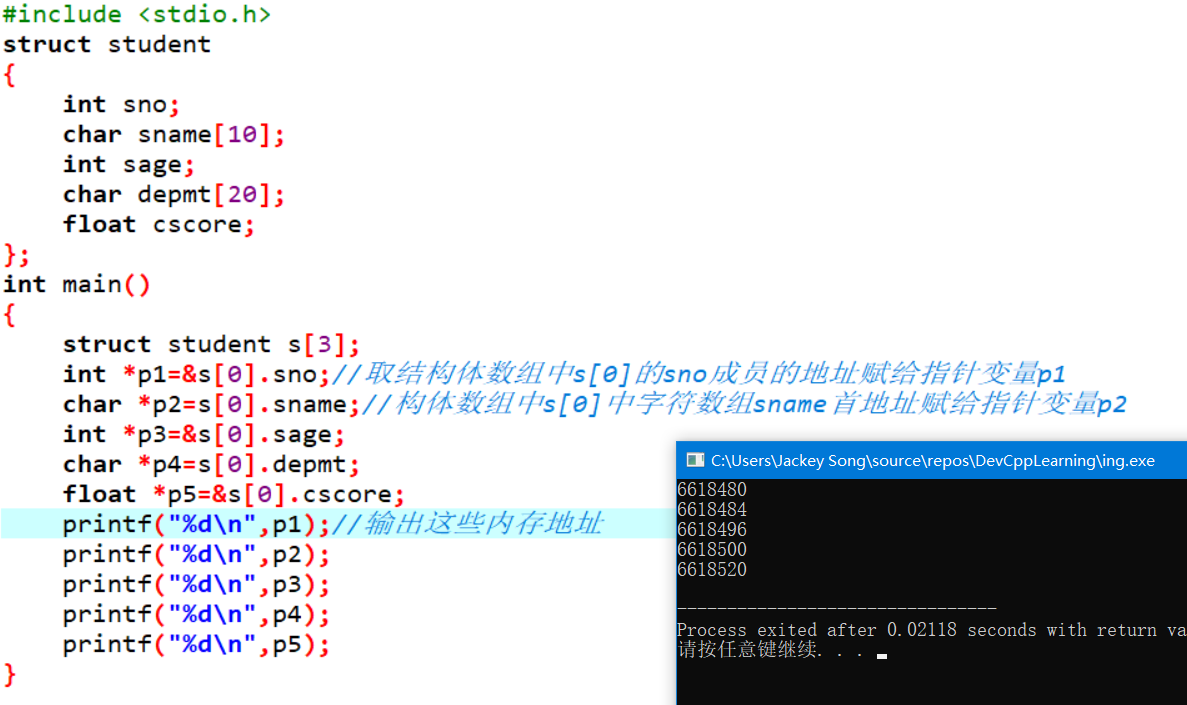内存地址相减得到各成员变量所占的字节数
注意： 访问结构体中的数据时，格式为结构体变量.结构体成员，这个时候使用的是点. 如果是一个指针变量p，p指向一个结构体类型，用指针来访问的话那么格式为指针变量->结构体成员，这个时候使用的是减号和大于号->，表示一个箭头，注意区分一下，使用的时候不要出错
结构体数组初始化
格式为：结构数组[n] = {{初值表1},{初值表2}, ... ,{初值表n}};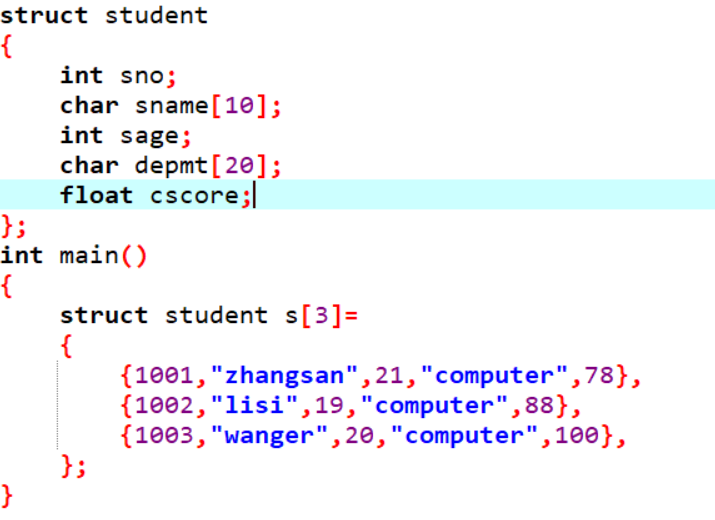用for循环输出结构体数组中的內容：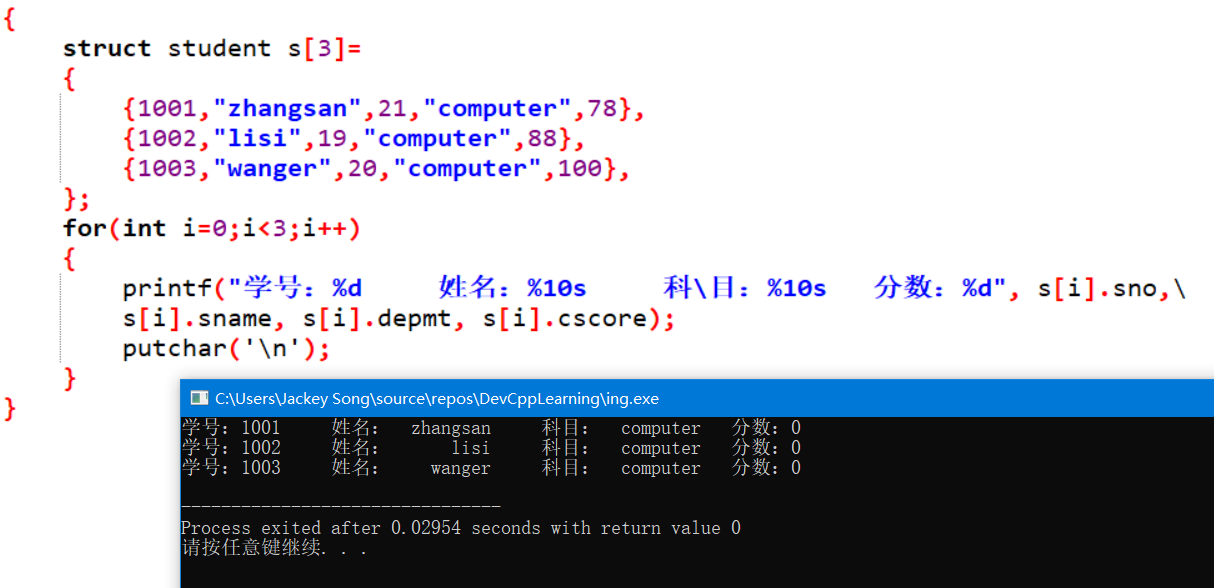当一个代码语句太长时，用反斜杠来进行代码换行 图中的代码有个字母打错了，导致最后的成绩输出全部是0，分数是float型的，而输出的时候却用了%d，应该用%f
结构体数组作为函数的参数
结构体数组作为函数的参数时，既可以作为形参，也可以作为实参，和普通数组一样。实参到形参的传递为地址传递。
查找符合条件的人数(一)
#include <stdio.h>
#define N 10
struct student
{
int no;
char name;
int score;
};
int fun(struct student s[],struct student b[],int m,int n);
void output(struct student x[],int n);
int main()
{
struct student s[N]=
{
{1001,"zhangsan",79},
{1002,"lisi",87},
{1003,"wanger",83},
{1004,"mazi",86},
{1005,"wangwu",89},
{1006,"wangliu",75},
{1007,"liwu",84},
{1008,"zhangwu",93},
{1009,"zhangqi",85},
{1010,"masan",97},
};
int m,n,k;
struct student a[N];
scanf("%d%d",&m,&n);
printf("The original data:\n");
output(s,N);
k=fun(s,a,m,n);
printf("The student that score in %d & %d is:\n",m,n);
output(a,k);
return 0;
}
int fun(struct student s[],struct student b[],int m,int n)
{
int i,k=0;
for(i=0;i<N;i++)
{
if(s[i].score>=m&&s[i].score<=n)
b[k++]=s[i];
}
return k;
}
void output(struct student x[],int n)
{
int i;
printf(" no          name       score\n");
for(i=0;i<n;i++)
printf("%d   %10s       %d\n",x[i].no,x[i].name,x[i].score);
putchar('\n');
}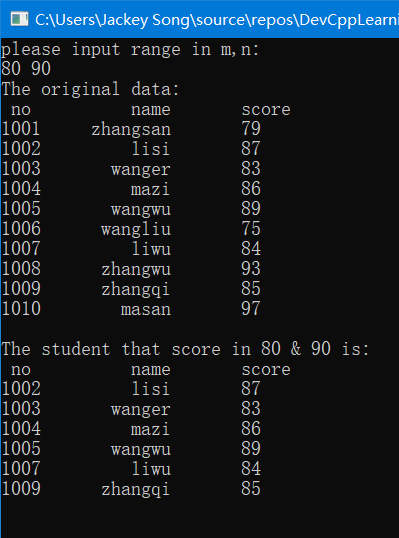结构体类型指针 和 指向结构体数组的指针
指向结构体变量的指针的定义
struct 结构体类型名 *指针名;
struct student stu;
struct student *ps=&stu;

利用结构体指针引用结构体变量成员
指针变量->结构体成员名; 取內容符*，*指针变量表示指针指向的变量， 也可以(*指针变量).成员名 所以有三种方法可以指向结构体成员变量： 结构体变量名 . 成员变量名 指向结构体变量的指针变量的名字 -> 成员变量名 (*指向结构体变量的指针变量的名字) . 成员变量名，括号不可省略
"."是分量运算符

指向结构体数组的指针
struct student s;
struct student *ps=s;//结构体变量名“s”为结构体数组的首地址

也可以写成：
struct student *ps=&s;

查找符合条件的人数(二)
结构体数组作为函数的实参时，可以用指针变量作为形参来接收结构体数组的内存地址，进而进行操作，把上面的代码改用指针来做，如下：(用指针做会更麻烦一些，但是为了加深对指针的印象和应用，我还是用指针做了一遍)
#include <stdio.h>
#define N 10
struct student
{
int no;
char name;
int score;
};
int fun(struct student *p,struct student *p2,int m,int n);//用指针来接收结构体数组的内存地址
void output(struct student *p,int n);//用指针来接收结构体数组的内存地址
int main()
{
struct student s[N]=
{
{1001,"zhangsan",79},
{1002,"lisi",87},
{1003,"wanger",83},
{1004,"mazi",86},
{1005,"wangwu",89},
{1006,"wangliu",75},
{1007,"liwu",84},
{1008,"zhangwu",93},
{1009,"zhangqi",85},
{1010,"masan",97},
};
int m,n,k;
struct student a[N];
scanf("%d%d",&m,&n);
printf("The original data:\n");
output(s,N);
k=fun(s,a,m,n);
printf("The student that score in %d & %d is:\n",m,n);
output(a,k);
return 0;
}
int fun(struct student *p1,struct student *p2,int m,int n)//用指针来接收结构体数组的内存地址
{
int i,k=0;
for(i=0;i<N;i++)
{
if((p1+i)->score>=m&&(p1+i)->score<=n)//用指针指向结构体数组中的人成员变量
*(p2+k++)=*(p1+i);//结构体变量之间的赋值
//注意k++和++k之间的区别
}
return k;
}
void output(struct student *p,int n)//用指针来接收结构体数组的内存地址
{
int i;
printf(" no          name       score\n");
for(i=0;i<n;i++)
printf("%d   %10s       %d\n",(p+i)->no,(p+i)->name,(p+i)->score);
putchar('\n');
}typedef类型
typedef的使用形式:typedef 标准类型名 别名; 如：
typedef int INTEGER;

为标准类型int定义一个别名，可以使用INTEGER来定义变量
INTEGER a,b;

结构体也可以使用typedef类型定义：
typedef struct student
{
int no;
char name;
int score;
}STU;

这样就可以使用STU来定义结构体变量：
STU s1,s2;
STU s;

学生成绩排序
输入n(n<=50)个学生的成绩信息，按照学生的个人平均成绩从高到低输出他们的信息。
#include <stdio.h>
struct student
{
int num;
char name;
int computer,english,math;
double average;
};
int main()
{
int i,index,j,n;
struct student stu,temp;

printf("Input n:");
scanf("%d",&n);
for(i=0;i<n;i++)
{
printf("Input the info of No.%d:\n",i+1);
printf("number:");
scanf("%d",&stu[i].num);
printf("name:");
scanf("%s",stu[i].name);
printf("math score:");
scanf("%d",&stu[i].math);
printf("english score:");
scanf("%d",&stu[i].english);
printf("computer score:");
scanf("%d",&stu[i].computer);
stu[i].average=(stu[i].math+stu[i].english+stu[i].computer)/3.0;
}
for(i=0;i<n-1;i++)
{
index=i;
for(j=i+1;j<n;j++)
if(stu[j].average>stu[index].average)
index=j;
temp=stu[index];
stu[index]=stu[i];
stu[i]=temp;
}
printf("num\tname\taverage\n");
for(i=0;i<n;i++)
printf("%d\t%s\t%.2lf\n",stu[i].num,stu[i].name,stu[i].average);

return 0;
}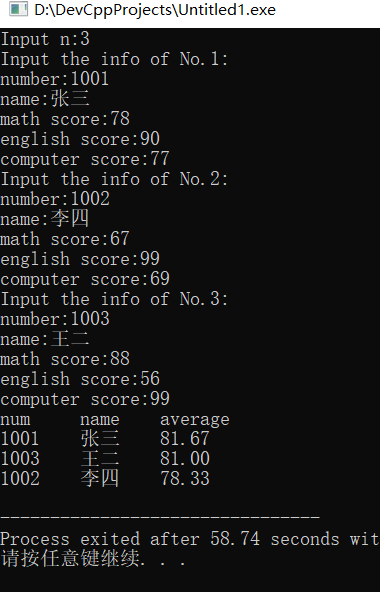修改学生成绩
输入n(n<=50)个学生的成绩信息，再输入一个学生的学号、课程以及成绩，在自定义函数中修改该学生指定课程的成绩
#include <stdio.h>
struct student
{
int num;
char name;
int computer,english,math;
double average;
};
int updata_score(struct student *p,int n,int num,int course,int score);
int main()
{
int course, i, n, num, pos, score;
struct student stu;
printf("Input n:");
scanf("%d",&n);
for(i=0;i<n;i++)
{
printf("Input the info of No.%d:\n",i+1);
printf("number:");
scanf("%d",&stu[i].num);
printf("name:");
scanf("%s",stu[i].name);
printf("math score:");
scanf("%d",&stu[i].math);
printf("english score:");
scanf("%d",&stu[i].english);
printf("computer score:");
scanf("%d",&stu[i].computer);
}
printf("Input the number of the students to be updata:");
scanf("%d",&num);
printf("Choise the course: 1.math  2.english  3.computer:");
scanf("%d",&course);
printf("Input the new score:");
scanf("%d",&score);
pos=updata_score(stu,n,num,course,score);
if(pos==-1)
else
{
printf("After updata:\n");
printf("num\tmath\tenglish\tcomputer\n");
printf("%d\t%d\t%d\t%d\n",stu[pos].num, stu[pos].math, stu[pos].english, stu[pos].computer);
}
return 0;
}
int updata_score(struct student *p,int n,int num,int course,int score)
{
int i,pos;
for(i=0;i<n;i++,p++)
if(p->num==num)
break;
if(i<n)
{
switch(course)
{
case 1:p->math=score;break;
case 2:p->english=score;break;
case 3:p->computer=score;break;
}
pos=i;
}
else
pos=-1;
return pos;
}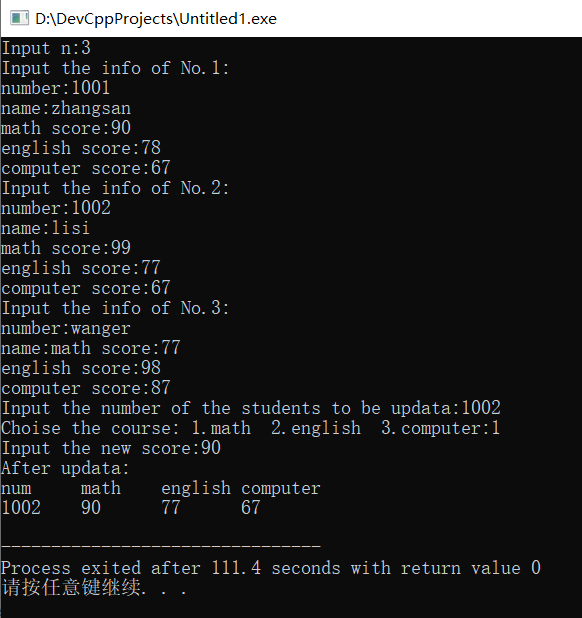下一篇文章
动态数组的实现，结合指针与结构体实现链表，以及链表相关操作
展开全文• 三个老师，每个老师带五个学生，老师是一个数组，每个老师带的五个学生又是一个数组。 #include <iostream> #include<string> using namespace std; //学生结构体 struct student{ string name; int ...c++
• 一、定义结构体数组，并初始化 //首先声明结构体类型 struct students { char name; int age; }; //定义结构体数组，并初始化 struct students stu={"Allen",18,"Smith",19,"Grace",18}; 为了提高代码...c语言 程序设计
• #include <string.h>///memset需要头文件<string.h>（c语言） 或 <cstring>（c++语言) struct node { int w1, w2, w3;...///结构体数组可以直接memset初始化 return 0; } ...
• ## 结构体数组的定义

千次阅读 2019-08-28 11:38:20
1、先定义结构体类型，再定义结构体数组 struct student { int num; char name; char sex; int age; }; struct student stu; 2、定义结构体类型的同时定义结构体数组 struct student { int num;...
• 结构体数组的初始化与前面讲的数值型数组的初始化是一模一样的，数值类型数组初始化的方法需要注意的是在结构体数组的初始化中同样适用，因为不管是不是数值型数组还是结构体数组都是数组。 #include<stdio.h>...
• 定义结构体数组定义结构体变量的方法相仿，定义结构体数组时只需声明其为数组即可。如： struct Student //声明结构体类型Student { int num; char name; char sex; int age; float score; char addr...c语言 数据结构
• 结构体定义与使用 结构体是一种构造数据类型 把不同类型的数据组合成一个整体 结构体定义形式： struct 结构体名{ 结构体所包含的变量或数组 }; 结构体是一种集合，它里面包含了多个变量或数组，它们的...C语言
• C#中结构体定义时一些注意事项 本篇文章主要为记录使用结构体时遇到的一些问题，方便以后查找。 C#中定义结构体主要形式为 using System.Runtime.InteropServices; //结构体属性（一字节对齐），不写的话默认4...c#
• c数组定义与初始化 int array;//长度100; int array = {1,2,3,4,5,5,6}//定义时进行初始化 int * a =0 ;//a指向int数据 int* array = new int//定义一个指向int类型数据的指针 int* array = new int...
• 结构体指针的定义方式如下： struct Student stu = { 1001,"Li Lei",'M',1.87 }; //定义一个Student 的结构体变量stu struct Student *p=&stu; //定义一个Student的结构体指针变量来指向stu 当程序中定义了...
• 当需要定义的参数变量较多时，往往需要用到结构体，Matlab中结构体数组与python中字典概念差不多，使用方法也类似，下面详细介绍其定义方法。 方法一： 直接定义，如定义 // An highlighted block students.name = '...Matlab
• 同普通变量和数组一样，需要多个变量的时候我们需要引入数组，如果要定义多个结构体，那么我们同样是要引入结构体数组结构体数组的本质也是一个一维数组，只不过一维数组的每一个成员又是结构体。 typedef struct ...
• struct student{ int num; char name;...结构体定义 student stud; 数组变量的定义 void input (student &stud){ cout<<"请输入xxx:"; cin>>stud...
• ## 结构体数组定义

千次阅读 2018-08-07 22:06:32
结构体数组定义：具有相同结构体类型的结构体变量组成的数组  定义结构体数组的方法和定义结构体变量的方法类似。 eg： #include&lt;stdio.h&gt; #include&lt;string.h&gt; #define N 10 ...
• #include <stdio.h> #include <stdlib.h> typedef struct sf { int n; char c;... //定义结构体sf，给他起个别名fc int main ( int argc, char *argv[] ) { int i...
• go语言结构体数组定义 介绍 (Introduction) Building abstractions around concrete details is the greatest tool that a programming language can give to a developer. Structs allow Go developers to describe...java python 编程语言 大数据 go
• MATLAB中定义结构体数组 最近使用MATLAB代码生成，结构体数组的问题困扰许久，先将支持代码生成的结构体数组创建方法总结如下，主要参考MATLAB帮助文档。 0. MATLAB中的结构体 结构体是使用被称为字段的数据容器将...matlab 数据结构
• 设一个结构体数组，数组中包含3个元素 每个元素中的信息应包括候选人的姓名(字符型)和得票数(整型) 输入被选人的姓名，然后与数组元素中的“姓名”成员比较，如果相同，就给这个元素中的“得票数”成员的值加1 输出...
•python 数据结构...